Refractive index profile calculation

38 views (last 30 days)
Tay on 15 May 2020
Commented: Rena Berman on 13 Dec 2021
Hello all,
I'm tryng to calculate a Refractive index profile from an article (Ref 1):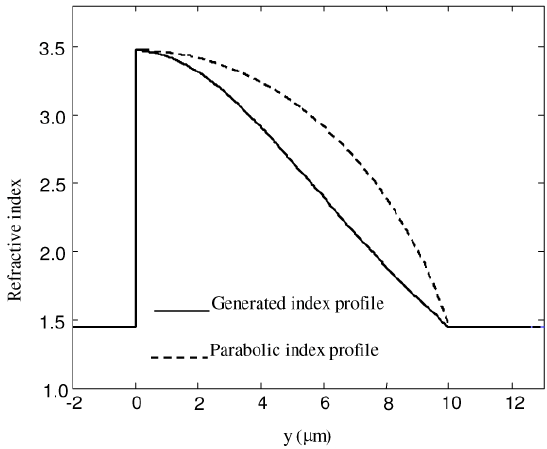But my code does not reproduce smothly as it was suppose to do. Goes to 1.48 (last value as the curve above) but as a square: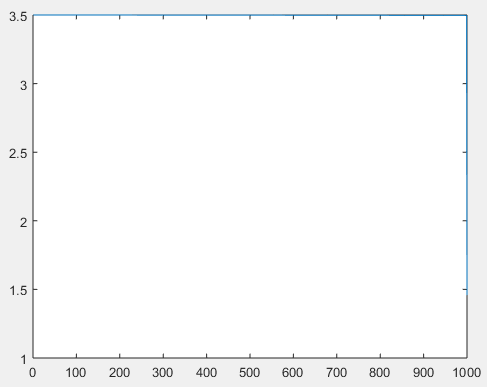The thing is that as you can see in my code below I have N layers (this means N values of refrective index) and when I change the values below 1000 I get some weird shapes. The right curve (first curve attached in this text) has 1000 of values, I mean they (arthurs of that curve) used N = 1000 because converge to a continuos curve. I think I'm very near to the response but I don't know what could be wrong.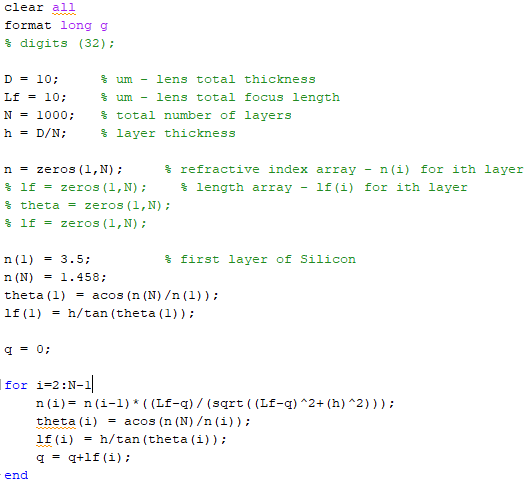If I change N = 10, for example, look the shape: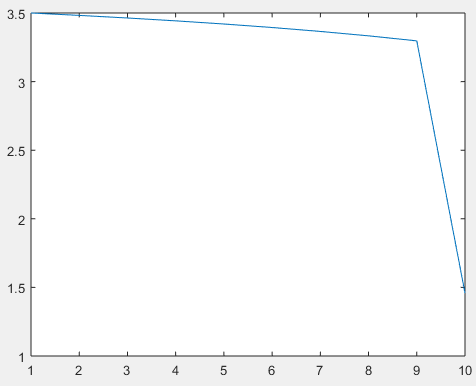I mean is not continuos, of course, because it is no the right value that the artours used to had that curve but with N = 1000 it is like a square. And I'm using all the equations to calculate right. Check this:
1. From Ref 1 they said: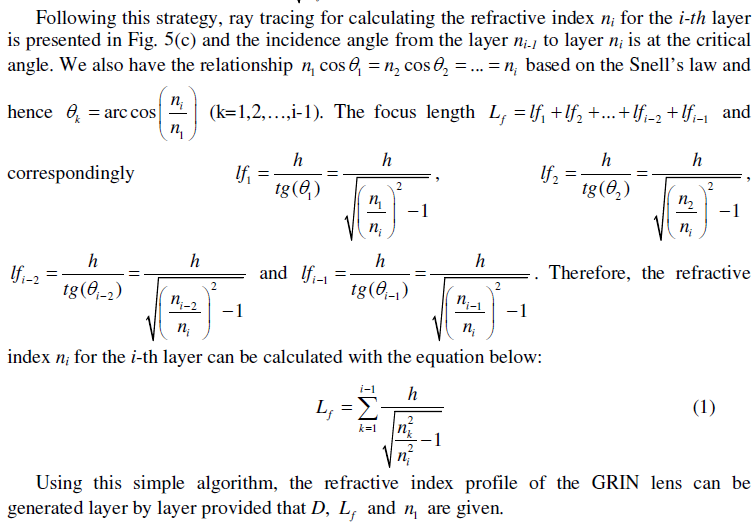2. And the figure that I'm studying to reproduce is (From Ref. 1):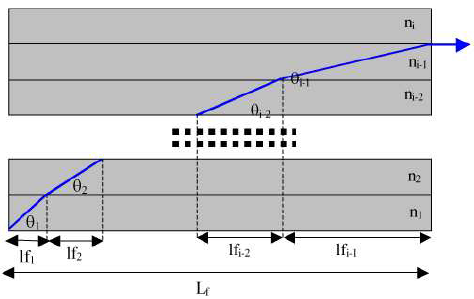As you can see in my code I calculate theta correct and also Lf up to Lf maximum (this case is 10um (as you can see in the article curve and in my code)).
I really appreciate any help !!
NOTE: THE ARTICLE HAS OPEN ACESS FROM OSA WEBSITE. IT IS NOT RESTRICTED SO ANYONE CAN SEE.
References
1. Qian Wang, Yingyan Huang, Ter-Hoe Loh, Doris Keh Ting Ng, and Seng-Tiong Ho, "Thin-film stack based integrated GRIN coupler with aberration-free focusing and super-high NA for efficient fiber-to-nanophotonic-chip coupling," Opt. Express 18, 4574-4589 (2010).
Rena Berman on 13 Dec 2021

David Goodmanson on 19 May 2020
Hi Tay,
The following code addresses (1) in the text. For Lf = 1, D = 1 and 1000 layers the resulting plot reproduces the one in the text pretty well.
When D > Lf you can get some funky results with the refractive index being less than 1.
Lf = 1;
D = 1;
N = 1000;
h = D/N;
Lfh = Lf/h;
n1 = 3.5; % refractive index of layer 1
nvec = n1; % vector of refractive indices
for k = 1:N
ni = fzero(@(ni) fun(ni,nvec,Lfh),[.1, nvec(end)-1e-10]); % have to be careful with upper limit
nvec = [nvec ni];
end
plot(nvec)
grid on
function y = fun(ni,nvec,Lfh)
y = sum(1./sqrt(nvec.^2/ni^2-1)) - Lfh;
end
Tay on 24 Nov 2021
Thank you David. this was supposed to be simple, but it started to be an forum
no problem, it's ok hahaha